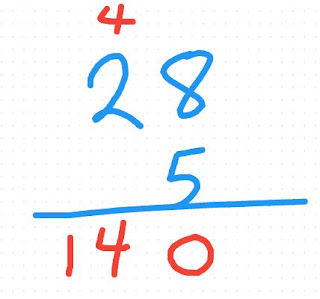Thursday, December 31, 2015

Mental Math and More Than One Way to Solve a Problem

Try this for your opener next time you have class.  Or better yet, at a social event.  This is fascinating.

No calculator, no talking, and no writing...

What is 5 times 28?

Give your students (or friends) a minute to think through their answer.  You might even want to tell them to confirm their answer using another method if the have finished.

Group your students randomly into threes.  ( I do this by taking my class size divided by 3 and rounding up to the nearest whole number.... If I have 26 in the class that day, divided by 3 rounded up is 9.   Count up to 9 over and over until all have a number.  1's get together, 2's get together and so on.  The 9's group will have only two people in it. )

Have each person discuss their solution and how they arrived at it.   Once the group has finished talking about how they solved it, have them try to find other ways to solve the question.

Now go back as a big group and discuss the different ways of solving the problem.

Here are some ways to solve this.  I'm sure there are many more too.

1.  5 times 8 is 40.  5 times 20 is 100.  Add 40 and 100 to be 140.  ( a great opportunity to talk about the distribution property)
2.  5 times 25 is 125.  5 times 3 is 15.  Add 125 and 15 to be 140.  (distribution property again)
3.  2 times 28 is 56.  Double that and get 112.  Add another 28 to get 140.
4.  10 times 28 is 280.  Take half of that to get 140.
5.  Take 28 and change it to be 14 times 2.  Now multiply the 2 and the 5 to be 10.  Then multiply the 10 and 14 to be 140.   ( I love the rearranging of factors to be helpful to multiply in other ways)
6.  Take 28 and change it to be 7 times 4.  Now multiply the 4 and the 5 to be 20.  Then multiply the 20 and 7 to be 140.
7.  I find many students go to doing this in their head.... See below.Isn't that cool?  So many different strategies.  Did you find a different one?  Please comment below.

Once you have discussed all the possibilities.  Ask your students if they would change their original strategy?  Why would you change?  I think it is important for our students to see that other students have different approaches to the same problem AND THEY ARE CORRECT TOO.

I think too often we as teachers give a certain method as THE answer and the students feel wrong if they have done it by another method.  This will help us all realize how important it is to justify what you do with words and explanations.

This exercise will help your students realize the value of mental math.  It will also help your students appreciate the amazing variety of answers.  I like to do this at least every other week in my class to help promote mental math strategies and an appreciation of other peoples methods of solving.

What do you think?